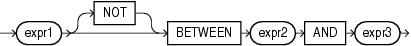## BETWEEN Condition

A `BETWEEN` condition determines whether the value of one expression is in an interval defined by two other expressions.

between_condition::=Description of the illustration between_condition.eps

All three expressions must be numeric, character, or datetime expressions. In SQL, it is possible that `expr1` will be evaluated more than once. If the `BETWEEN` expression appears in PL/SQL, `expr1` is guaranteed to be evaluated only once. If the expressions are not all the same data type, then Oracle Database implicitly converts the expressions to a common data type. If it cannot do so, then it returns an error.

The value of

```expr1 NOT BETWEEN expr2 AND expr3
```

is the value of the expression

```NOT (expr1 BETWEEN expr2 AND expr3)
```

And the value of

```expr1 BETWEEN expr2 AND expr3
```

is the value of the boolean expression:

```expr2 <= expr1 AND expr1 <= expr3
```

If `expr3` < `expr2`, then the interval is empty. If `expr1` is `NULL`, then the result is `NULL`. If `expr1` is not `NULL`, then the value is `FALSE` in the ordinary case and `TRUE` when the keyword `NOT` is used.

The boolean operator `AND` may produce unexpected results. Specifically, in the expression `x AND y`, the condition `x IS NULL` is not sufficient to determine the value of the expression. The second operand still must be evaluated. The result is `FALSE` if the second operand has the value `FALSE` and `NULL` otherwise. See Logical Conditions for more information on `AND`.

Table 6-10 BETWEEN Condition

Type of Condition Operation Example
`[NOT] BETWEEN x AND y`

[`NOT`] (`expr2` less than or equal to `expr1` `AND` `expr1` less than or equal to `expr3`)

```SELECT * FROM employees
WHERE salary
BETWEEN 2000 AND 3000
ORDER BY employee_id;```